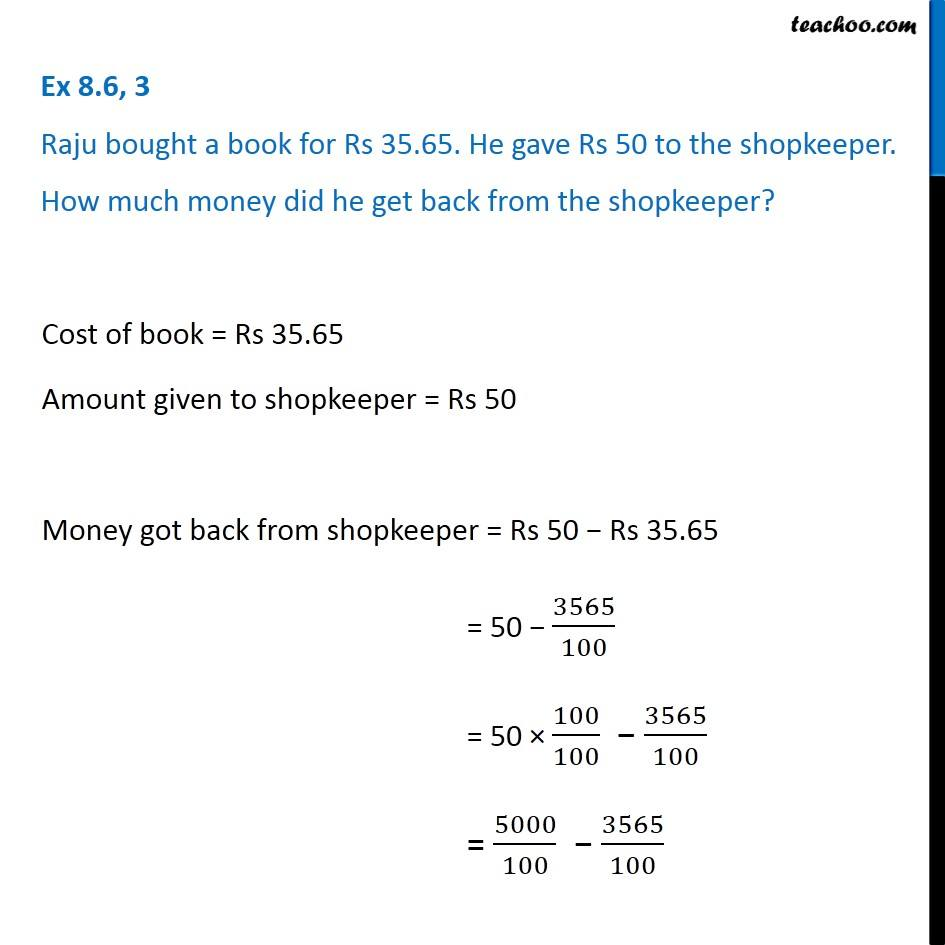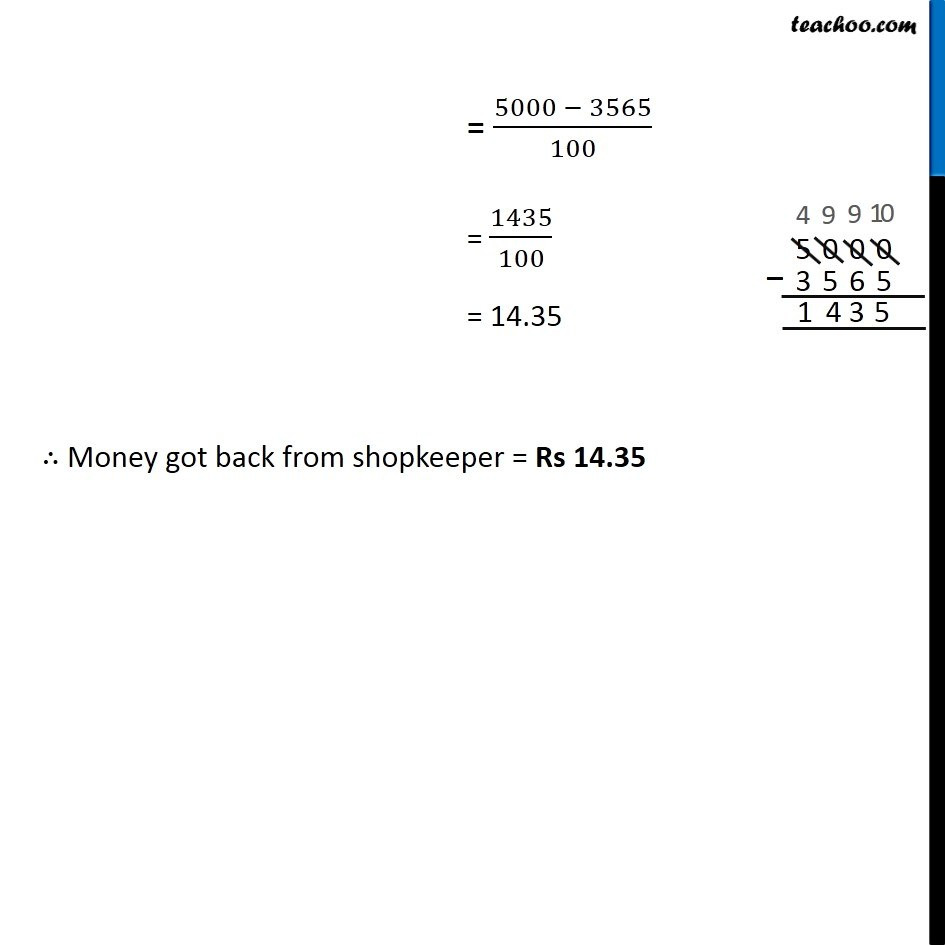Subtraction of decimal numbers - Statement questions

Chapter 8 Class 6 Decimals
Concept wise### Transcript

Ex 8.6, 3 Raju bought a book for Rs 35.65. He gave Rs 50 to the shopkeeper. How much money did he get back from the shopkeeper? Cost of book = Rs 35.65 Amount given to shopkeeper = Rs 50 Money got back from shopkeeper = Rs 50 − Rs 35.65 = 50 − 3565/100 = 50 × 100/100 − 3565/100 = 5000/100 − 3565/100 = (5000 − 3565)/100 = 1435/100 = 14.35 ∴ Money got back from shopkeeper = Rs 14.35